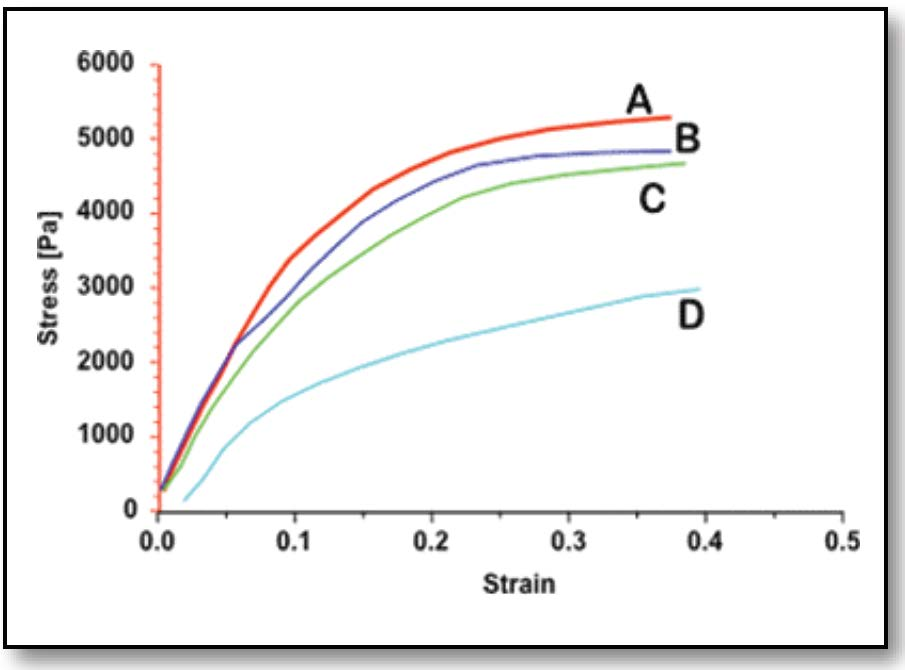Skip to contentUSE
For linoleum and other types of heavy duty flooring materials.

ISSUE
Heavy flooring adhesives generally produce highly variable viscosity test results when using standard viscometers. RST-SST Rheometer in a yield test with modulus calculation (slope of line leading up to yield stress) quickly qualifies the product with consistently repeatable results, thereby providing an improved QC procedure.

TEST EQUIPMENT
Instrument: RST-SST Soft Solids Tester
Geometry: VT-20-10 Vane Spindle
Software: RHEO 3000

TEST METHOD
Shear Rate (CSR) control with vane spindle rotating at a low speed.

PROGRAM
• One step
• Start RPM = 0.5
• End RPM = 0.5
• Time = 30 seconds
• Plot = Stress on Y-axis, Strain on X-axis
• Regression analysis using values up to 40% of maximum shear stress

Four types of adhesives are evaluated in Figure 1. Modulus determination was set to evaluate up to 40% of peak stress value. The following modulus values were reported:

Sample A: G = 35550 Pa
Sample B: G = 34419 Pa
Sample C: G = 28862 Pa
Sample D: G = 16652 Pa

The vane spindle geometry easily accommodates adhesives of varying consistency and produces a repeatable curve that allows for quick pass/fail test. In Figure 1, Sample D has the lowest modulus and therefore spreads most easily. Samples A and B have the highest modulus and are comparatively stiffer when spreading.Figure 1: Evaluation of Four Types of Adhesives
Stress vs. Strain